# Chapter 9 Circles NCERT Exemplar Solutions Exercise 9.3 Class 10 Maths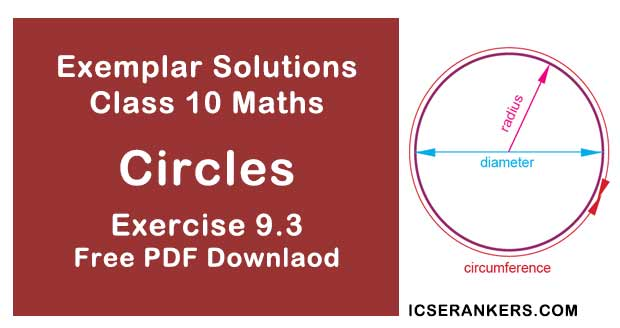Chapter Name NCERT Maths Exemplar Solutions for Chapter 9 Circles Exercise 9.3 Book Name NCERT Exemplar for Class 10 Maths Other Exercises Exercise 9.1Exercise 9.2Exercise 9.4 Related Study NCERT Solutions for Class 10 Maths

### Exercise 9.3 Solutions

1. Out of the two concentric circles, the radius of the outer circle is 5 cm and the chord AC of length 8 cm is a tangent to the inner circle. Find the radius of the inner circle.

Solution

Let C1 , and C2  be the two circles having same centre O. AC is a chord which touches C1 at point D.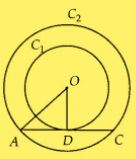Join OD.
Also, OD ⊥ AC
AD = DC = 4 cm
[Perpendicular line OD bisects the chord]
In right angled ∆AOD,
OA2 = AD2 + OD2 [By Pythagoras theorem]
⇒ OD2 = 52 – 42
⇒ OD2 = 25 – 16 = 9
⇒ OD = 3 cm
Radius of the inner circle is OD = 3 cm

2. Two tangents PQ and PR are drawn from an external point to a circle with centre O. Prove that QORP is a cyclic quadrilateral.

Solution

We know that,
Radius ⊥ Tangent = OR ⊥ PR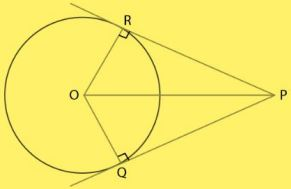∠ORP = 90°
Similarly,
Radius ⊥ Tangent = OQ ⊥PQ
∠OQP = 90°
Sum of all interior angles = 360°
∠ORP + ∠RPQ+ ∠PQO + ∠QOR = 360°
⇒ 90° + ∠RPQ + 90° + ∠QOR = 360°
So,
∠O + ∠P = 180°

3. If from an external point B of a circle with centre O, two tangents BC and BD are drawn such that ∠DBC = 120°, prove that BC + BD = BO, i.e., BO = 2BC.

Solution

As given in the question,
By RHS rule,
ΔOBC and ΔOBD are congruent {By CPCT}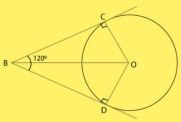∠OBC and ∠ OBD are equal
Therefore,
∠OBC = ∠OBD =60°
In triangle OBC,
cos 60°= BC/OB
⇒ 1⁄2 = BC/OB
⇒ OB = 2BC
Hence proved.

4. Prove that the centre of a circle touching two intersecting lines lies on the angle bisector of the lines.

Solution

Let us take the lines be l1 and l2 .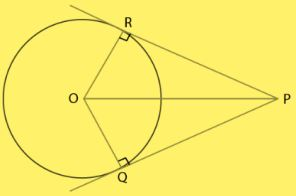Assume that O touches l1 and l2 at M and N,
So,
OM = ON  (Radius of the circle)
Therefore,
From the centre ”O” of the circle, it has equal distance from l1 & l2.
In Δ OPM & OPN,
OM = ON  (Radius of the circle)
∠OMP = ∠ONP  (As, Radius is perpendicular to its tangent)
OP = OP  (Common sides)
Therefore,
Δ OPM = ΔOPN  (SSS congruence rule)
By C.P.C.T,
∠MPO = ∠NPO
So, l bisects ∠MPN.
Hence, O lies on the bisector of the angle between l1 & l2 .
Also,
Centre of a circle touching two intersecting lines lies on the angle bisector of the lines.

5. In Fig. 9.13, AB and CD are common tangents to two circles of unequal radii. Prove that AB = CD.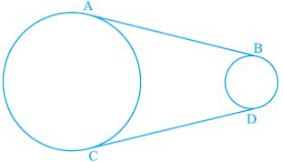Solution

As given in the question,
AB = CD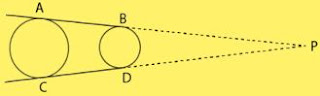Construction: Produce AB and CD, to intersect at P.
Proof:
Consider the circle with greater radius.
Tangents drawn from an external point to a circle are equal
AP = CP ...(i)
Also,
Consider the circle with smaller radius.
Tangents drawn from an external point to a circle are equal
BP = BD ...(ii)
Subtract Equation (ii) from (i),
AP – BP = CP – BD
⇒ AB = CD
Hence Proved.

6. In Question 5 above, if radii of the two circles are equal, prove that AB = CD.

Solution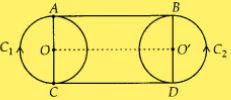Join OO’
Since, OA = O’B [Given]
And,
∠OAB = ∠O’BA = 90°
[Tangent at any point of a circle is perpendicular to the radius at the point of contact]
Since, perpendicular distance between two straight lines at two different points is same.
AB is parallel to OO’
Also,
CD is parallel to OO’
AB || CD
Now,
∠OAB = ∠OCD = ∠O’BA = ∠O’DC = 90°
ABCD is a rectangle.
Hence,
AB = CD.

7. In Fig., common tangents AB and CD to two circles intersect at E. Prove that AB = CD.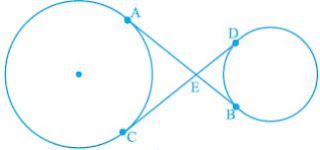Solution
Given, common tangents AB and CD to two circles intersecting at E.
To prove:
AB = CD
We have,
The length of tangents drawn from an external point to a circle is equal
EB = ED and
EA = EC
EA + EB = EC + ED
⇒ AB = CD

8. A chord PQ of a circle is parallel to the tangent drawn at a point R of the circle. Prove that R bisects the arc PRQ.
Solution
Given, chord PQ is parallel to tangent at R.
To prove : R bisects the arc PRQ.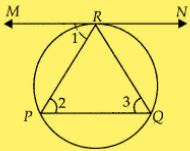∠1 = ∠2   [Alternate interior angles]
∠1 = ∠3
[Angle between tangent and chord is equal to angle made by chord in alternate segment]
∠2 = ∠3
PR = QR  [Sides opposite to equal angles are equal]
As,
arc PR = arc QR
Therefore, R bisects PQ.

9. Prove that the tangents drawn at the ends of a chord of a circle make equal angles with the chord.
Solution
To prove : ∠1 = ∠2
Let RQ be a chord of the circle. Tangents are drawn at the points R and Q.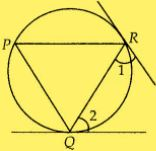Let P be another point on the circle, then join PQ and PR.
As, at point Q, there is a tangent.
∠2 = ∠P [Angles in alternate segments are equal]
Also, at point R, there is a tangent.
∠1 = ∠P [Angles in alternate segments are equal]
⇒ ∠1 = ∠2 = ∠P
⇒ ∠1 = ∠2

10. Prove that a diameter AB of a circle bisects all those chords which are parallel to the tangent at the point A.
Solution
Given, AB is a diameter of the circle.
A tangent is drawn at point A.
Draw a chord CD parallel to tangent MAN.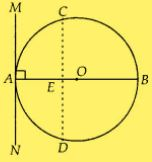Therefore, CD is a chord of the circle and OA is radius of the circle.
∠MAO = 90°
[tangent at any point of a circle is perpendicular to the radius through the point of contact]
∠CEO = ∠MAO  [Corresponding angles]
⇒ ∠CEO = 90°
So,
OE bisects CD,
[Perpendicular from centre of circle to chord bisects the chord]
Similarily,
Diameter AB bisects all chords which are parallel to the tangent at the point A.